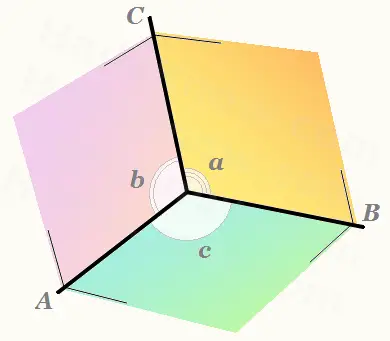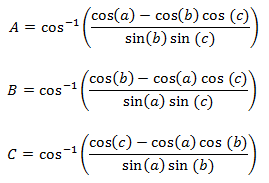# Dihedral Angle Calculator for Polyhedra

Dihedral Angle Calculator
Input vetex angles, output dihedral angles
a = o
b = o
c = o
A =
B =
C =

If three faces meet at a vertex on a polyhedron and you know the three vertex angles, then you can use formulas from spherical trigonometry to compute the angles between two adjacent faces, the dihedral angle.

In the figure below, the vertex angles are labeled a, b, and c, while the dihedral angles are labeled A, B, and C. (In spherical trigonometry, the subtending angles of a solid angle correspond to a, b, and c, while the surface angles correspond to A, B, and C.)

You can use the calculator on the left to compute the dihedral angles for any degree-three vertex, for example, in a tetrahedron. Some dihedral angles of common polyhedra are given in the table below.Polyhedron Exact Dihedral Angle Dihedral Angle in Degrees PLATONIC Regular Tetrahedron cos-1(1/3) 70.52878° Cube 90° 90° Octahedron cos-1(-1/3) 109.47122° Dodecahedron cos-1(-1/√5) 116.56505° Icosahedron cos-1(-√5/3) 138.18969° Cuboctahedron cos-1(-1/√3) 125.26439° Rhombic Dodecahedron 120° 120° Rhombic Triacontahedron 144° 144°